# 9.00 Data Preprocessing

Preprocessing is an essential part of creating machine learning models. Preprocessing is typically used to convert data to an appropriate type, to normalize the data in some way, or to extract useful features. In previous chapters, most preprocessing operations were done automatically by the tools we used, but in many cases, it has to be done separately. In this chapter, we will review classic preprocessing methods that are important to know about.

### Preprocessing Pipeline

Let’s start by training a classifier on the Titanic survival problem:

Let’s now extract the internal feature preprocessor that the automatic function came up with:

This represents a chain of preprocessors, which is called a preprocessing pipeline. Some of these preprocessors are structural, such as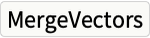, which simply merges vectors into a single vector. Other preprocessors contain learned parameters, such as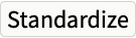, which learned the means and variances of numeric variables. Each preprocessor can be seen as a simple model that learns from the data. The preprocessors are learned and applied to the data one preprocessor at a time.

In this case, Classify first created the preprocessor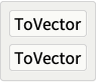and applied it to the training data. It then proceeded to learn the preprocessor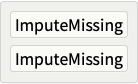from the resulting data and applied it again, and so on for the rest of the preprocessors, such as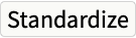etc.

Once the preprocessing is done, we need to keep the preprocessing pipeline as part of the model in order to apply it to new data. A classic mistake is to preprocess the training data and then forget about it. This can lead to very bad performance on unseen data. The training data and in-production data should always be processed in the exact same way. This is also valid if we import a model; we need to make sure that we use the preprocessor that led to the training data of this model.

### Numeric Data

Most variables are numeric. Here are classic preprocessing techniques applied to numeric data.

#### Standardization

A classic preprocessing step is to standardize the data, which means setting the mean of each variable to 0 and the standard deviation to 1. Standardization is an important first step of many applications because it allows for the ignoring of the scale of variables. For example, if a standardization is performed, it would not matter if a length is expressed in meters or in centimeters. Let’s show this on the variable "Age" from the Titanic Survival dataset:

There are ages between 0 and 80 years old. Let’s estimate their mean:

Let’s now estimate their standard deviation, which is the root mean square of the centered data:

From these values, we can create a processing function that can standardize this data and future data as well:

Most libraries have tools to obtain such a preprocessor in one step, such as:

Let’s compare the original and standardized values:

Standardized values are centered and their magnitudes are around 1. If ages were expressed in months, here it would not matter. Note that the two histograms have the same shape; we just shifted and contracted the data.

Standardization is a very common preprocessing method. Many machine learning methods expect the data to be standardized. That said, if we know that variables are similar in nature (same unit, same scale), we should probably not standardize them. For example, we would not standardize pixel values in a dataset of images.

#### Log Transformation

Often, the numeric values of a variable are of the same sign and span many orders of magnitude. We saw it in the brain/body weight dataset:

A mole weighs 122 grams while a Brachiosaurus weighs 87 tons, which means that their weights differ by six orders of magnitude. Let’s visualize a histogram of these weights:

We can barely see anything because all of the values are small compared to the scale set by the Brachiosaurus. Most methods and algorithms cannot handle such heavy-tailed distributions. In the vast majority of cases, it is better to preprocess the data so that the values have the same scale. We can do this by applying a log transformation. Let’s visualize the resulting histogram.

Now values are next to each other, they have the same scale, and the histogram looks more like a Gaussian distribution. Preprocessing a variable like this is also an implicit way to express that we care more about ratios than differences for this variable.

#### Discretization

Another classic preprocessing method is data discretization, also called binning, which consists of converting a numeric variable into a categorical variable. This can, for example, be needed if we want to use a machine learning method that requires categorical data.

Let’s show discretization preprocessing using the ages of Titanic passengers:

We can see that these ages are already a bit discretized since most of them are rounded to the year. Let’s reduce the number of categories further.

The idea is to partition the space by defining intervals, like a histogram would. Each interval, often called a bin, corresponds to one category. We could define the intervals ourselves, such as "Baby" for ages below two years old, "Children" for ages between two and 10 years old, etc. Such a human discretization could give good results since we inject a bit of our knowledge about the world here. More commonly, discretization is learned automatically from the data. There are plenty of methods to do this. We could simply divide the space into equal parts. We could also find intervals that contain the same number of values, which are called quantiles. Here is what we would obtain by using quantiles in order to have 100 ages in each bin:

The intervals would then be:

Such quantile discretization is often better than using equally sized bins because it is robust to any reversible transformation of the data (e.g. squaring the ages before a quantile discretization has no consequence on the result).

#### Dimensionality Reduction

Dimensionality reduction is often used as a preprocessing method to speed up subsequent computations, to reduce memory, or to improve model quality. We will not detail this preprocessing method since it is presented in Chapter 7, Dimensionality Reduction.

#### Missing Data Synthesis

Many machine learning methods cannot handle missing values, so a very common preprocessing step is to fill them in by predicting what these values should be. See Chapter 7, Dimensionality Reduction, and Chapter 8, Distribution Learning, for more details.

### Categorical Data

Most applications require data to be numeric. Here are the usual transformations used to convert categorical variables ("Cat", "Dog", etc.) to numeric variables.

#### Integer Encoding

The simplest way to transform a categorical variable into a numeric one is to assign a different integer value to each category, such as:

Let’s apply this index to some values:

One issue with such an integer encoding is that we added an irrelevant distance relation between classes (there is no reason to have "Bird" closer to "Dog" than to "Cat"). In a sense, we lost the information that categories are different things. Also, the resulting space is unidimensional, which can make it hard for machine learning methods to work with. For all these reasons, integer encoding is generally considered a bad preprocessing method unless it is followed by a one-hot encoding or another kind of vector embedding.

#### One-Hot Encoding

The solution to avoid the shortcomings of integer encoding is to map the categorical variable to a multidimensional space, which means transforming each category into a vector of numeric values. One way to do this is to use one-hot vectors. One-hot vectors are bit vectors that are 0 everywhere except for one value. Here is an example: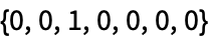For our categorical variable, the encoding would be:

Let’s apply this index to some values:

The space is now multidimensional, and we did not introduce artificial relations between categories. They are all equivalent. One extra advantage of this encoding is that there is one variable per category, which is useful for interpreting simple models in the field of statistics.

One-hot encoding is a classic and heavily used preprocessing method. However, one important issue with this encoding is that it returns large sparse vectors when there are many categories. Many machine learning methods (such as a decision tree) are not adapted to such data and they generate models that do not generalize well.

#### Vector Embedding

One-hot encoding replaces categorical values with one-hot vectors, but we could also use other numeric vectors. The generic term for this is vector embedding.

We could, for example, use a random embedding, which uses a random vector for each category. Here is an example of such an embedding:

Let’s apply this index to some values:

Note that even if the vectors are random, the same category is always encoded by the same vector.

Such randomly generated preprocessing might appear foolish, but it works well in practice when the number of variables is large and the embedding dimension is not too small. One thing to understand is that if the embedding dimension is large enough, random vectors will be at about the same distance from each other. The advantage of this embedding over a one-hot encoding is that we can control the embedding dimension (we chose 4 here). For example, if we have 1000 variables, choosing a dimension such as 50 will probably work better than the 1000 dimensions imposed by a one-hot encoding. This dimension can be determined through a validation procedure.

There are various ways to achieve the same kind of encoding using deterministic vectors. One way is to use the numbers generated by a Hadamard matrix:

This avoids the storing of random vectors and might work better for small dimensions.

An even better alternative is to learn an encoding from the data. This is what neural networks usually do with categorical variables: they include an embedding step that is jointly learned with the rest of the network. Here is an embedding layer that can take three categories (which should be integer encoded beforehand) and returns vectors of size 4:

This layer stores one numeric vector per category, and the vectors are learned during the training. Here is the initial encoding applied to our classes (equivalent to the random encoding):

It is also possible to learn an encoding using a neural network on a task and then to reuse this encoding for another downstream task, which is a form of transfer learning.

### Image

Image processing is a rich field. Let’s review the main image preprocessing and feature extraction methods used in machine learning.

#### Image-to-Image Processing

As a first step, we might want to modify images. We can, for example, reduce their resolutions to save memory and time for subsequent tasks:

Another classic step is to conform every image to have the same shape, color space, type of encoding, etc., such as:

This step is required by most machine learning methods.

We can also perform classic operations such as adjusting brightness, contrast, etc.:

More importantly, we can blur, rotate, add noise, or randomly crop images for data augmentation purposes, which is very frequently done before training a neural network:

These transformations allow for the injection of the knowledge we have about this data (e.g. that a rotated bird is still a bird).

Finally, we can also apply existing machine learning models as a preprocessing step, such as extracting objects or faces:

There are plenty of other image-to-image processing methods that we could perform. Nowadays, such processing tends to be limited to the operations covered here though, as we typically let neural networks learn for themselves what to do.

#### Pixel Values

Machine learning methods require numbers as input. One way to transform an image into numbers is simply to extract its pixel values:

This is an array (or tensor in neural network terms) that has three dimensions (the array is said to be of rank 3). One dimension is for the width of the image, one is for the height, and one is for the colors. For some machine learning methods, such as convolutional neural networks (see Chapter 11, Deep Learning Methods), this is all we need. For more classic methods, we might want to flatten all these numbers into a single vector:

#### Classic Feature Extraction

Pixel values encode all the information contained in an image, but it is not easy to obtain semantic information from pixel values (e.g. the objects, their relations, etc.). To make things easier, we can try to extract features from images.

Historically, all sorts of features from the field of signal processing have been used for machine learning applications. A simple type of feature is statistics about colors:

Here we could use the mean, standard deviation, etc. of these histograms as features. We can also try to find where edges are using classic algorithms:

Similarly, there are a variety of algorithms to find shapes or keypoints such as:

Another classic feature extraction method uses Gabor filters to detect textures:

Here the filter detects regions for which intensity varies along the horizontal axis at a given frequency. Similarly, we can use things like Fourier transforms and wavelet transforms to extract features:

These types of features used to be dominant in the field of machine learning before the rise of neural networks. Nowadays, these features are still used when we cannot use a neural network, such as in small embedded devices.

#### Neural Feature Extraction

The current best way to extract semantic features from an image is to use a neural network. We saw an example of this in Chapter 3, Classification, and in the Transfer Learning section of Chapter 2. The idea is to use a neural network trained on a generic task, such as image classification, and to extract the features it produces. For example, here is a network trained to recognize about 4000 classes:

This network is a chain of layers, each computing a numeric representation of the image from the previous numeric representation, starting with pixel values. We can use the output of any layer as features. Generally, we use one of the last layers to obtain semantically rich features. Here are about 1000 features obtained after the 22 nd layer:

Such features are useful for many tasks.

The majority of networks used as feature extractors are first trained to classify images, but some are trained on other tasks such as colorizing images, predicting image depth, detecting objects, or even synthesizing missing parts of images.

### Text

Text is another kind of data that requires preprocessing in order to be useful.

#### Text-to-Text Processing

The first thing we generally want to do is to put text in some canonical form. A common procedure is to lower the casing of every character:

This allows strings such as "The" and "the" to be understood as the same thing. A similar transformation removes diacritics:

Diacritics are pretty rare in English but are common in other languages, and since they are often omitted, it is usually better to just remove them. There are other things we might want to canonicalize such as font variants, circled variants, width variants, fractions, and various marks:

This is called text normalization, and we used the normalization form KD here. Such procedures are very common before any natural-language processing task.

#### Tokenization

After normalization, the usual next step is to tokenize the text document, which means breaking it into substrings called tokens or sometimes terms. Tokens can simply be characters:

The resulting tokens form a categorical variable, and we could encode them with integers such as:

Here are our tokens integer encoded:

The set of all possible tokens is called a vocabulary, which we chose to be the letters of the alphabet here. Out-of-vocabulary tokens can be deleted or replaced by a default value.

Character tokens have the advantage of letting models learn without many assumptions. Such models can find relations between similarly spelled words or understand misspelled or made-up words from their spelling. Also, when the task is about generating text (like for translation), characters allow for the generation of any possible string.

The problem with characters is that models need to learn everything from scratch. It is often more data efficient to use a higher-level semantic unit such as words:

After tokenization, these words are categorical values. Their original string does not matter anymore and we could integer encode them as well: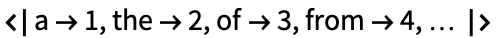Learning from words typically requires less data to obtain good results than learning from characters. However, one issue with words is that they are not well defined, especially in some languages. Also, word tokenization loses spelling information. For example “fish” and “fishing” would be considered two completely different things. Similarly, out-of-vocabulary words, such as misspelled or made-up words, are completely ignored. Finally, this tokenization can lead to very large vocabularies (up to millions of words), which can cause issues.

A more modern and usually better alternative is to use a subword tokenization. This method uses characters, words, and also word parts (subwords) as tokens. These tokens are learned from a corpus. The basic idea is that rare words should be split into subwords, but frequent words should not. A classic method to learn such tokenization is using an adaptation of the byte pair encoding compression algorithm. Here is a subword tokenizer learned from a large amount of text using this method:

This tokenizer learned 3000 different tokens (this number is a hyperparameter that can be changed), and they have quite different lengths. Here is a sample of these tokens:

We can see that characters, subwords, and full words are included. The tokenizer extracts tokens iteratively from left to right by picking the longest matching token each time. Here is a visualization of tokens extracted from a piece of text:

Usual words such as “the” or “moon” are tokenized entirely, while rarer and more complex words such as “satellite” are decomposed. Also, out-of-vocabulary words are not discarded; they are tokenized into known subwords, including characters if necessary:

Because of these properties, subword tokenization is dominant in modern natural-language processing applications.

#### TF–IDF & Latent Semantic Indexing

After tokenization, each document is a sequence of categorical values. We sometimes need to transform these sequences into fixed-size feature vectors. This would be the case when using the logistic regression or the random forest method, for example. It could also be the case when defining a distance between documents for information retrieval purposes. We can achieve this by computing count vectors. Let’s say that we want to encode the following set of documents (called a corpus in natural-language processing terms):

We can compute the vocabulary contained in these documents:

To create a count vector, we simply need to count the number of occurrences of each token in a document:

Now every document is a numeric feature vector with the number of features equal to the size of the vocabulary. Note that the position of tokens in the document does not matter anymore. We lost this information. This is called a bag-of-words assumption (even though tokens might not be words). This set of vectors is called a term-document matrix.

Such count vectors are not so much used in this form. One issue is that common words have a lot of importance in these vectors. Usually, a better solution is to compute term frequencyinverse document frequency vectors, more commonly known as tfidf vectors, instead. The idea is to weight tokens depending on their rarity. Let’s compute the tfidf vectors for our example. We first need to compute term (i.e. token) frequencies, which are just token counts normalized by the number of tokens in the document:

We now need to compute the document frequency for each token, which is the fraction of the document in which this token is present:

Then we just need to multiply the term frequencies by the log of the inverse of these document frequencies:

And that’s it. As we can see, the token “the,” which is present in every document, now has a value of 0 everywhere, so it is effectively ignored. On the other hand, the words that are seen in only one document have a higher weight that others. Such vectors are well suited for information retrieval (i.e. search engines).

The usual next step after obtaining a count matrix or tfidf matrix is a linear dimensionality reduction. Indeed, term-document matrices are sparse (lots of zeros), so they are hard to manipulate and are not handled well by most machine learning methods. It would be better to compress these long sparse vectors into short dense ones. As it happens, linear dimensionality reduction can be performed very efficiently on sparse data. Let’s train a dimensionality reducer on our tfidf matrix:

Documents are now represented with only three numeric values:

Working with such reduced and dense vectors should be fast, and we generally obtain better results using these vectors than using the original sparse vectors. The procedure of computing term-document matrices and reducing them linearly is called latent semantic analysis, and it is particularly used for information retrieval (it is also called latent semantic indexing in this context).

#### Word Vectors

As we have seen before, image classification makes heavy use of transfer learning. In its simplest form, a features extractor is trained on a large dataset and then used on the target dataset to convert images to vectors. Can we do the same with text?

Assuming that we tokenize with words (the following is valid for any kind of tokenization), one solution is to use word vectors, a.k.a. word embeddings. The idea is to learn a vector representation for each possible word on a large dataset and use them as features. The learning task to obtain these vectors could be to predict the following word given the current word. Using our previous corpus, this would mean learning from the following supervised dataset:

This is a simple classification task, and we could use a shallow linear network to tackle it such as:

Note that the first layer is an embedding layer that stores one vector per word. This is the layer that we are interested in. Let’s train this network on our classification data:

We now just need to extract the vectors inside this embedding layer and associate them with their tokens:

Let’s visualize these words in their embedding space:

Even with such a tiny training set, we can already see that grammatically similar words tend to be next to each other. With more data, both grammar and semantics would be captured by such a procedure. This is because similar words tend to be used in similar ways.

We can now use these learned embeddings to encode new documents:

Such processed documents contain grammatical and semantic information about their words instead of raw tokens, so it will be easier to learn from them. This is a form of transfer learning.

There are several methods to learn word embeddings, which are all leveraging the fact that similar words are used in the same context. Famous methods include Word2Vec (which uses a prediction task like we discussed previously) and GloVe (which performs a dimensionality reduction on a word co-occurrence matrix). Here are word embeddings learned by GloVe from a dataset of six billion words and 400000 unique words:

Each word is mapped to 50 dimensions:

Here is a visualization of some of these vectors with their dimensions reduced to two:

Again, similar words are placed next to each other.

An interesting property of some of these learned vector spaces is that some directions encode human concepts, such as what a capital city is. For example, the learned representation can figure out that France is to Paris as Germany is to Berlin just by adding and subtracting the corresponding vectors:

These analogy completions via vector arithmetic are a sign that these embeddings form a good representation.

Note that after transforming tokens into vectors, documents still have variable sizes. This is why we need to use methods that can handle sequences of vectors after this preprocessing, such as recurrent neural networks (RNN) or transformer networks (see Chapter 11, Deep Learning Methods). This can be problematic when the data is very small because these methods do not typically work well on tiny datasets.

Such word vectors (and token vectors in general) are simple to learn, are easy to use, and can radically improve the performance of machine learning applications. This word-vector preprocessing used to be the standard procedure for most natural-language processing applications until the rise of contextual word vectors, which will be presented in the next section.

#### Contextual Word Embeddings

The main limitation of word vectors as presented earlier is that they are not context dependent. Most words have a different grammatical function or a different meaning depending on their context. For example, the word “Amazon” can refer to a river, a company, or a mythical nation of female warriors; it could probably refer to other things as well. These ambiguities are one of the main problems of natural-language understanding, and traditional word vectors do not solve this for us.

In order to capture the meaning of a text, one solution is to embed a complete sentence instead of a single word, which means transforming each sentence into a fixed-size numeric vector. While intuitive, this approach is (currently) not favored, one reason being that sentence lengths can vary so a fixed-size solution is not ideal.

A better solution is to use contextual word embeddings. Each word (or token more generally) is transformed into a vector, but this time the vector also depends on the context. We can obtain such a feature extractor by training a neural network on a word-prediction task such as:

This is similar to training traditional word vectors, but this time, we will use vectors computed deeper in the network instead of the vectors learned in the first layer. Let’s implement a naive model to obtain such vectors. We will use a Sherlock Holmes book as a training set and tokenize it:

To simplify things, let’s only predict the next word given past words, and set the data as a classification task:

We only predict from the last five words here to simplify things further, but the history could be as long as we want and even of varying size. Let’s now define a neural network for this task:

This network has an embedding layer (from which we could obtain traditional word vectors, but we are not interested in that) and then a long short-term memory layer (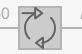), which is a type of recurrent layer (see Chapter 11, Deep Learning Methods). Recurrent layers take a sequence of vectors as input and return another sequence of vectors as output. It is this last sequence of vectors that interests us. Let’s train this network:

We can use the trained network to generate Sherlock-like text, which is not great (we would need more training data):

Let’s now transform this network into a feature extractor by taking the first two layers:

This extractor transforms each word into a vector of 50 numeric values:

Because these vectors are used to predict the next word, they contain information about the current word but also previous ones; they can be considered contextual word embeddings. Note that the definition of contextual word embeddings is not very clear since each vector could contain all kinds of information about other words that are not necessarily related to the given word.

There are better methods to learn contextual word embeddings. A classic one is called BERT. Here is a BERT model trained on books and Wikipedia:

This model has been trained on more than two billion words on tasks such as predicting missing tokens. Also, it uses a transformer architecture, which happens to work better than recurrent networks for text (see Chapter 11, Deep Learning Methods). Each token (which is a subword here) is converted into a vector of 768 values:

These vectors depend on the context. Here is an illustration where we color each token according to their embeddings (we reduce the dimension of the vectors from 768 to 3 to make them RGB colors):

We can see that when the word “Amazon” corresponds to the river, it is colored in green while it is colored in black or dark red when it corresponds to the company.

These contextual word embeddings are heavily used to tackle natural-language processing applications. The usual workflow is to use a pre-trained model (such as BERT) that has been trained on a large corpus by someone else because training such a model from scratch can be very expensive. Then, if our data is small, we can just compute the vectors and learn on top of them. If our data is large and diverse enough, we can also fine-tune the word embeddings model to our task to improve performance further. Note that in both cases, we need to use a machine learning method that can handle sequences of vectors, such as a neural network with a convolutional, recurrent, or transformer architecture.

Takeaways
 ■ Preprocessing can be used to convert variables to a given type, normalize data, or extract features. ■ Preprocessors can learn from data. ■ Preprocessors are typically assembled into a preprocessing pipeline. ■ The preprocessing pipeline should be integrated into the downstream model and applied to new data. ■ Standardization is the main preprocessing for numeric variables. ■ Categorical variables are usually transformed into numeric vectors. ■ Tokenization is a necessary step to preprocess text. ■ Image, text, and audio need to be converted into numeric or categorical data. ■ Neural networks can be used to extract semantic features from images, text, and audio.
Vocabulary
Numeric Preprocessing
Categorical Preprocessing
 integer encoding encoding each category by an integer one-hot vectors vectors that are 0 everywhere except for one value that is 1 one-hot encoding encoding each category by a one-hot vector vector embedding encoding categorical values into numeric vectors random embedding vector embedding using a random vector for each category
Image Preprocessing
 image conformation transform every image so they have the same shape, color space, type of encoding, etc. data augmentation procedure to augment the number of image examples by applying classic transformations to the images (e.g. blurring, rotating, cropping, etc.) arraytensor data structure for which elements are indexed by a multidimensional position; vectors and matrices are arrays of dimensions 1 and 2, respectively array rank dimensions of an array signal processing engineering subfield that focuses on processing signals such as audio, images, and scientific measurements Fourier transform preprocessing that extracts the periodic components of spatial or temporal data neural feature extraction extracting semantic features using a neural network semantic features features that capture the meaning of data examples
Text Preprocessing
Exercises
9.1Synthesize missing values from the Titanic Survival dataset, then standardize the numeric variables. Implement a function to perform both operations on unseen data.
9.2Generate numeric values that have heavy tails in both directions using a Cauchy distribution. Try to find a preprocessing method to obtain data that looks more like it comes from a normal distribution.
9.3Transform every example of the Titanic Survival dataset into a numeric vector.
9.4Train classifiers on 100 MNIST images using different feature extractors. Possible feature extractors include pixel values, Fourier transforms, the ImageIdentify network, and the LeNet network. Compare their results.
9.5Create a program to learn a byte pair encoding tokenization.
9.6Create a “search engine” to find the most similar sentence to a query using BERT as a preprocessor. Try it on some Wikipedia pages.